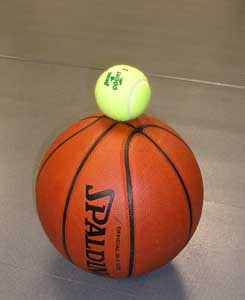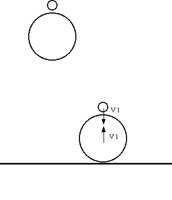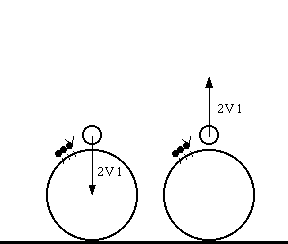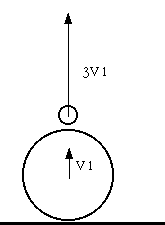Bouncing Balls

Gaining HeightMaterial

• A tennis ball

To Do and Notice

Drop the basketball and notice how high it bounces,
it will certainly bounce up to a height lower than the height from which you dropped it.

Drop the tennis ball, it too bounces up to a height lower than that from which it was dropped.

Now hold the tennis ball directly above the basketball so that the balls are touching.

Drop both balls at the same time.

Notice that the tennis ball goes shooting off above your head at a high speed.Drop two balls, one above the other. They hit the floor and the bottom ball reverses direction colliding with the upper ball.

What's Going On?

Consider a ball bouncing off the floor.

You drop the ball and it accelerates down pulled by gravity.

If the center of the ball drops by one meter it hits the floor with a speed about V1 = 4 m/s.

When the ball hits the floor the ball deforms, it becomes a squashed spring which pushes down on the floor. In return the floor pushes up on the ball slowing its downward speed at first and then accelerating it back up. If no energy is lost as heat when the ball deforms, then the ball leaves the floor heading up at the same speed with which it hit the floor, 4 m/s. It then rises to the same height from which it was dropped. If any energy is lost then the ball leaves the floor at a slower velocity and rises to a lower height.

The ratio of the speed after the collision, va, to the speed before the collision, vb, is called the coefficient of restitution. COR = vb/va.

The weird thing about all this is that the ball bounces off the floor because the floor pushes it up.

Now consider two balls.

The lower basketball behaves as described above. Bouncing upward off the floor at V1 = 4 m/s. The tennis ball is still falling at V1 = 4 m/s.

Let's move into the fame of reference of an ant sitting on top of the basketball. Relative to the earth, the ant is traveling upward at 4 m/s, but the ant is at rest relative to the basketball. The ant looks up and sees a tennis ball coming down at 2V1 = 8 m/s.This is just like driving down a highway doing 100 km/hr, you look at the cars approaching you also doing 100 km/hr relative to they highway, they are traveling 200 km/hr relative to you.

The tennis ball bounces off the basketball. It comes in at 8 m/s and it bounces up at 2V1 = 8 m/s. (It behaves just like any ball bouncing off the floor, which is really just the bigger ball of the earth.) Now let's leave the ant and look at the situation after the tennis ball has bounced off the basketball from the frame of reference of the earth. The tennis ball is going upward 8 m/s faster than the basketball, but in the earth frame the basketball is going upward at 4 m/s so the tennis ball is going upward at 3V1 = 12 m/s = 8 m/s + 4 m/s.This is a general result, when a light ball is dropped onto the floor on top of a heavy ball and no energy is lost to heat then the light ball will bounce upward at three times the velocity with which it would have hit the floor if dropped alone.

This becomes even more impressive when you realize how high the light ball will bounce. The maximum height of a ball thrown straight upward occurs when the ball stops at the top of its trajectory. All of its energy is potential energy, E = mgh where g is the acceleration of gravity, m is the mass of the ball and h is the height. The energy as the ball leaves the floor is kinetic energy, E = 1/2 mv2 . When this kinetic energy is converted to potential energy then 1/2mv2 = mgh, and h = 1/2 v2/g. So the height is proportional to the velocity squared. Thus the tennis ball could go up to nine times higher than the height from which it is dropped.

Going Further

This experiment can also be done with three balls, a basketball, a tennis ball and a Ping-Pong ball. Go through the chain of reasoning for the three ball collision and find out the speed of the Ping-Pong ball.

This experiment can also help you understand gravity assisted spacecraft trajectories, in which a light spacecraft is "bounced" off a heavy planet.

You can also try dropping the basketball on top of the tennis ball. Notice that collisions can also act to remove energy from a ball.

This frames of refernce pdf will guide you through an analysis of the changing frames of reference.

Etc.

This experiment has also been done with raw eggs instead of tennis balls. this greatly adds to the excitement.